#### You may also like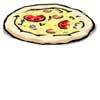### Pizza Portions

My friends and I love pizza. Can you help us share these pizzas equally?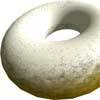### Doughnut

How can you cut a doughnut into 8 equal pieces with only three cuts of a knife?### Pies

Grandma found her pie balanced on the scale with two weights and a quarter of a pie. So how heavy was each pie?

# Fraction Lengths

## Fraction Lengths

Here are some lengths, which could be made out of connecting cubes or strips of coloured paper/card: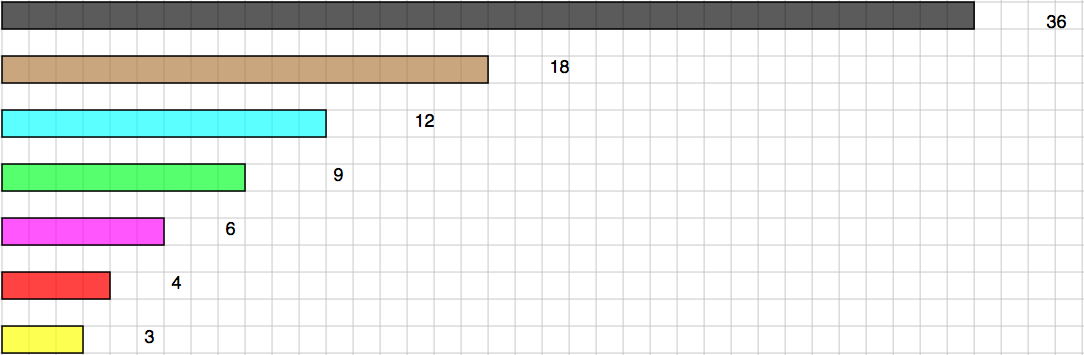To start with, the black will be counted as ONE so that the brown one is $\frac{1}{2}$, the blue one is $\frac{1}{3}$, etc.

Using different combinations, put them together to equal the length of the black, which is 36 long.
For example, if you were to choose the brown, blue and magenta (pink) you could write them down as the $\frac{1}{2}$, $\frac{1}{3}$, $\frac{1}{6}$

So we would have:   $\frac{1}{2} + \frac{1}{3} + \frac{1}{6} = 1$

- - - - - - - - - - - - - - - - - -

#### MOVING ON

Choose any four of the strips, except the black one, and put them together.
Now, compare them with the black.

Here are two examples to start you off. Have a go and find as many different fours as you can.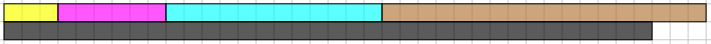Using a 3, 6, 12 and an 18 makes 1$\frac{1}{12}$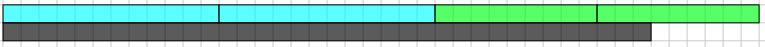Using two 12s and two 9s makes 1$\frac{1}{6}$

- - - - - - - - - - - - - - - - - -

#### GOING EVEN FURTHER

Now the light blue strip is the ONE (1).
Use the same fours that you chose before but this time, compare them with the light blue strip instead of the black.

Here are the examples used above, but this time compared with a light blue: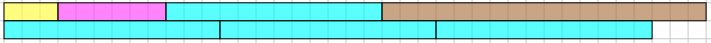Comparing these four to the light blue it makes 3$\frac{1}{4}$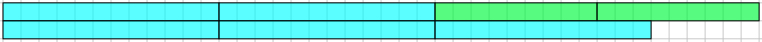Comparing these four to the light blue it makes 3$\frac{1}{2}$

Now you go ahead with the fours that you have chosen.

- - - - - - - - - - - - - - - - - -

What can you say about the results you got when comparing your fours with black and comparing them with the light blue?

### Why do this problem?

This activity gives the opportunity for meaningful discussion as learners find solutions so they will be deepening their understanding of the concept of fractions and becoming acquainted with relationships between fractions.  This activity also involves addition of fractions with different denominators and mixed numbers, so that the concept of equivalent fractions is also explored. As children tackle this task, it will be very important to repeatedly draw their attention to what is the "one".

### Possible approach

This activity can be introduced by showing the image on an IWB.  To begin with, you could say very little apart from asking the children what they notice or what they see.  This provides a non-threatening environment in which they can talk to a partner first and then share their 'noticings' with the wider group.

You could then introduce the first task.  Groups of pupils should have use of connecting cubes or strips of paper (till roll paper works well) or whatever is available to match the shown lengths.

There may be disagreements between different groups and this can lead to a healthy discussion as each group tries to justify their suggestions.  Try to step back as much as possible during this kind of discussion and remain a facilitator, so that the children look to each other for explanations, rather than straight to you.

### Key questions

Why is what you've chosen true?
Can you think of a way of writing that down? Can that be written a different way?

### Possible extension

If a group or individual pupil has successfully completed the third part of the task, then ask them to look at each fraction and relate it to the other sizes, for example:
The 6 is $\frac{1}{6}$ if the 36 is 1 whole
and is $\frac{1}{3}$ if the 18 is 1 whole
and is $\frac{1}{2}$ if the 12 is 1 whole
and is $\frac{2}{3}$ if the 9 is 1 whole
and is 1$\frac{1}{2}$ if the 4 is 1 whole
and is 2 if the 3 is 1 whole

You could also encourage similar comparisons when the compared length is not a whole 1 but, for example, $\frac{2}{3}$:
The 6 is $\frac{1}{9}$ if the 36 is $\frac{2}{3}$
and is $\frac{2}{9}$ if the 18 is $\frac{2}{3}$
and is $\frac{1}{3}$ if the 12 is $\frac{2}{3}$
and is $\frac{4}{9}$ if the 9 is $\frac{2}{3}$
and is 1 if the 4 is $\frac{2}{3}$

### Possible support

Some pupils may need reminding that the 36 is a whole 1 one all the time in the first part of the task.